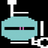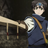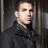首页   注册   登录
V2EX = way to explore
V2EX 是一个关于分享和探索的地方

V2EX  ›  程序员

# [c 语言或 Linux ] 吐槽加请教，高手请进

fuchar · 2018-11-19 20:01:40 +08:00 · 2023 次点击

• Return an array of arrays of size *returnSize.
• Note: The returned array must be malloced, assume caller calls free(). */ int cmp(int *a, int *b){ return *a - *b; }

int** threeSum(int* nums, int numsSize, int* returnSize) { int sum; int i, l, r, x, t=0; sum = (int)malloc(sizeof(int*)20000); /* 这是我原来的分配方式 * sum=(int)malloc(sizeof(int)200003); * for(i=1; i<20000; i++) * sum[i] = sum + i*3; **/ qsort(nums, numsSize, sizeof(int), cmp);

``````for(i=0; i<numsSize-2 && nums[i]<=0 ; i++ ){
if( i>0 && nums[i]==nums[i-1] )
continue;
for(l=i+1, r=numsSize-1; l<r; ){
x= nums[i]+nums[l]+nums[r];
if( x==0 ){
``````

//这是leetcode默认的分配方式 sum[t] = (int*)malloc(sizeof(int)*3); sum[t] = nums[i]; sum[t] = nums[l]; sum[t] = nums[r]; t++; for(r--; r>l && nums[r]==nums[r+1]; r--) ; for(l++; l<r && nums[l]==nums[l-1]; l++) ; } else if( x>0 ) for(r--;r>l && nums[r]==nums[r+1]; r--) ; else for(l++; l<r && nums[l]==nums[l-1]; l++) ; } } *returnSize = t; return sum;

}

11 回复  |  直到 2018-11-20 10:07:58 +08:001 Ediacaran   2018-11-19 20:12:16 +08:00 看描述好像是混淆了 指针的数组 和 二维数组 两个概念。2 raynor2011   2018-11-19 20:17:26 +08:00   ♥ 1 直接贴题目链接和代码吧，一大堆文字太难受了3 fuchar   2018-11-19 20:24:11 +08:00 @Ediacaran 可能是我没说清楚，函数要求返回一个 int**的指针。另外这个指针的问题我已经解决了，主要是想问有没有比较好的内存分配方式，既让 leetcode 主函数 free 成功，又可以提升效率，需要加一个数组的时候再用 malloc 分配空间，确实很耗时间4 2pang   2018-11-19 20:25:28 +08:00 via iPhone lz 你代码写错了你文中的 int** returnArry=(int**)malloc(10sizeof(int)int*的大小和 int 的大小不一定一样 也许你本地是 32 位 碰巧没出错5 fuchar   2018-11-19 20:32:28 +08:00 @2pang 笔误，笔误https://leetcode.com/problems/3sum//** * Return an array of arrays of size *returnSize. * Note: The returned array must be malloced, assume caller calls free(). */int cmp(int *a, int *b){ return *a - *b;}int** threeSum(int* nums, int numsSize, int* returnSize) { int **sum; int i, l, r, x, t=0; sum = (int**)malloc(sizeof(int*)*20000); /** *这是我原来的分配方式 * sum=(int*)malloc(sizeof(int)*20000*3); * for(i=1; i<20000; i++) * sum[i] = sum + i*3; qsort(nums, numsSize, sizeof(int), cmp); for(i=0; i0 && nums[i]==nums[i-1] ) continue; for(l=i+1, r=numsSize-1; ll && nums[r]==nums[r+1]; r--) ; for(l++; l0 ) for(r--;r>l && nums[r]==nums[r+1]; r--) ; else for(l++; l6 wevsty   2018-11-19 21:53:55 +08:00 基于 C99 标准考虑的话，要动态分配内存有两种办法1、可变长数组（ Variable length array，简称 VLA ）。2、malloc 系列函数。然而似乎很多编译器不支持 VLA，或者直接把 VLA 用 malloc 实现。所以从实际结果看，除了 malloc 以外没有什么其他方法动态分配内存。程序运行慢多半还是楼主自己实现的问题。* Return an array of arrays of size *returnSize.* Note: The returned array must be malloced, assume caller calls free().但是人家题目已经说的很明白了，这算是人家题目的要求，无视要求是无法得到一个正确答案的。7 contmonad   2018-11-20 00:25:54 +08:00 没啥简单的办法，他的接口已经设计成这样了。除非你知道 leetcode 用的哪种编译器 / malloc 算法，然后自己 hack 一下把元数据字段填上，骗过 free 调用。但是也不一定可以。8 ryd994   2018-11-20 01:33:45 +08:00 via iPhone 不要小看内存分配器你这种情况，即使是多次分配，很可能还是连续的9 iceheart   2018-11-20 06:10:18 +08:00 via Android 题目说的不是很清楚了吗？返回指针数组，每个元素用 malloc 分配。你究竟要搞什么事情？10 zwh2698   2018-11-20 06:34:29 +08:00 via Android C 语言是需要修炼的，不是学习可以解决的11 jmc891205   2018-11-20 10:07:57 +08:00 别在 leetcode 上 换个其他的用文本做输入输出的 OJ 刷题自己负责 free 就没这个问题了